In :
import plotly
import pandas as pd
import numpy as np
import seaborn as sns
import plotly.express as px

import matplotlib
import matplotlib.pyplot as plt
assert matplotlib.__version__ == "3.1.0","""
Please install matplotlib version 3.1.0 by running:
1) !pip uninstall matplotlib
2) !pip install matplotlib==3.1.0
"""

%matplotlib inline

In :
from IPython.core.display import display, HTML
display(HTML("<style>.container {width:90% !important;}</style>"))

In :
data = pd.read_csv('combined_set.csv')

In :
data['Mean Log GDP per capita']  = data.groupby('Year')['Log GDP per capita'].transform(
pd.qcut,
q=5,
labels=(['Lowest','Low','Medium','High','Highest'])
).fillna('Lowest')

In :
print(f'total number of missings vals: {data.isnull().sum().sum()} out of {data.shape * data.shape}')

total number of missings vals: 228 out of 36024

• Life Ladder respondents measure of the value their lives today on a 0 to 10 scale (10 best) based on Cantril ladder
• Log GDP per capita GDP per capita is in terms of Purchasing Power Parity (PPP) adjusted to constant 2011 international dollars, taken from the World Development Indicators (WDI) released by the World Bank on November 14, 2018
• Social support Answer to question: “If you were in trouble, do you have relatives or friends you can count on to help you whenever you need them, or not?”
• Healthy life expectancy at birth life expectancy at birth are constructed based on data from the World Health Organization (WHO) Global Health Observatory data repository, with data available for 2005, 2010, 2015, and 2016. To match this report’s sample period, interpolation and extrapolation are used.
• Freedom to make life choices Answer to question: “Are you satisfied or dissatisfied with your freedom to choose what you do with your life?”
• Generosity Responses to “Have you donated money to a charity in the past month?” compared to GDP per capita
• Perceptions of corruption Answer to “Is corruption widespread throughout the government or not?” and “Is corruption widespread within businesses or not?”
• Positive affect comprises the average frequency of happiness, laughter and enjoyment on the previous day.
• Negative affect comprises the average frequency of worry, sadness and anger on the previous day.
• Confidence in national government self explanatory
• Democratic Quality how democratic is a country
• Delivery Quality How well a country delivers on
• Gapminder Life Expectancy Life expectancy from Gapminder
• Gapminder Population Population of country

# Intro¶

I started learning Python more seriously about two years ago. Since then rarely a week has passed where I did not marvel at the simplicity and ease of use of Python itself or one of the many amazing open source libraries in the ecosystem. The more commands, patterns and concepts I become familiar with, the more everything just makes sense.

The opposit holds true for plotting with Python. Initially almost every chart I created looked like a crime escaped from the eighties. What makes matters worse is that to create said abonominations most of the time I had to spend hours on Stackoverflow researching nitty-gritty commands to change the slant of the x-ticks or something similiar silly. Don't even get me started on multi charts. While the results often looks fairly impressive and it is wonderful to create those charts programatically (e.g. 50 in a row), it is just so much work.

For a brief glimps in time I thought Bokeh >Link< would become my goto solution. I came accross Bokeh when I was working on geospartial visualizations. However, I quickly realized that Bokeh, while different, was just as stupidly complicated as matplotlib.

I did try out plot.ly a while ago while, again, working on visualization of geospartial data. Back that it seemed even more stupid than the afforementioned libraries. You needed an account and everything was rendered online and you would then download it. I quickly discarded plot.ly.

Ultimately I settled on using Pandas native plotting for quick inspections and Seaborn for charts for reports and presentations (where visuals matter). However, recently I watched a Youtube video about plotly express, where most importantly they got rid of all this online nonsese. I played around with it and must say, this might actually change my plotting life for the better.

In the following article, I will talk about:

• my general approach to visual data exploration on a conceptual level
• basic plotting with pandas
• advanced plotting with Seaborn
• creating beautiful advanced plots with plotly

## General approach¶

I tought statistics (Stats 119) whilst studying at Universtity in San Diego. Stats 119 is an into class to statistics. The curiculum included statistical fundamentals like data aggregation (visual and quantitative), concepts of odds and probabilities, regression, sampling, and - to me the most important one - distributions. This was the time my understanding of quantities and phenomena almost entirely shifted to a representation through distributions (most of the time Guassian).

To this day I find it astonishing how far the two quantities mean and standard deviation can get you in really grasping a distribution, the meaning and its implications.

By knowing these two numbers it is straightforward to conclude how likely a certain outcome is, one immediately knows, where the bulk of the results are going to be. It gives you a framework of reference to distinguise anecodatal events from statistically significant ones.

In :
np.exp(data[data['Year']==2018]['Log GDP per capita']).plot(
kind='hist'
)

Out:
<matplotlib.axes._subplots.AxesSubplot at 0x12b6a47f0>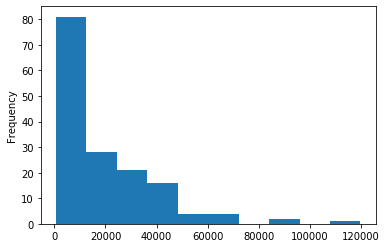In :
data['Year'].plot(
kind='hist',
figsize=(17,6),
title='Number of countries (y-axis) with certain nubmer of observations (x-axis)',
xlim=(2000,2025), # makes no sense
ylim=(2,50), # makes no sense
)

Out:
<matplotlib.axes._subplots.AxesSubplot at 0x1318a57b8>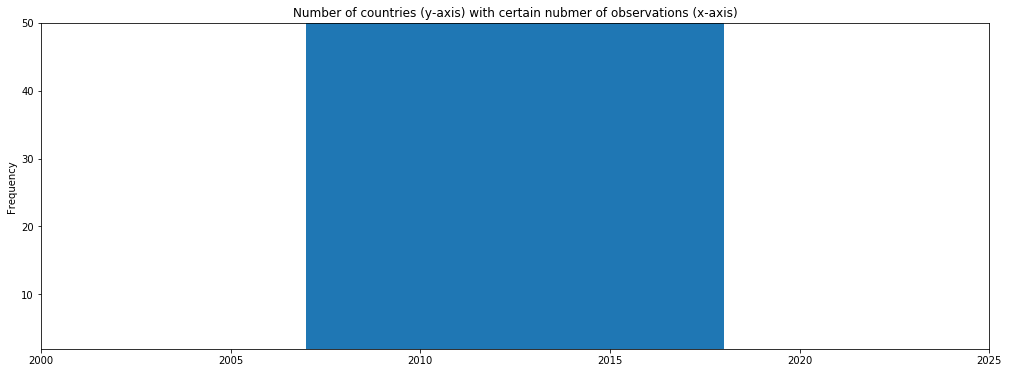In :
data[data['Year'] == 2018]['Life Ladder'].plot(
kind='hist'
)

Out:
<matplotlib.axes._subplots.AxesSubplot at 0x130d40668>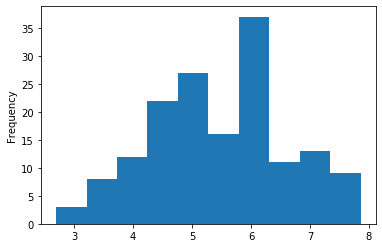In :
data[data['Year'] == 2018]['Life Ladder'].plot(
kind='hist',
bins=np.arange(2,8,0.25)
)

Out:
<matplotlib.axes._subplots.AxesSubplot at 0x1333aa080>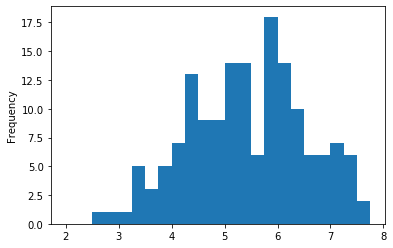In :
data[
data['Year'] == 2018
kind='bar',
figsize=(12,8)
)

Out:
<matplotlib.axes._subplots.AxesSubplot at 0x1332f2f98>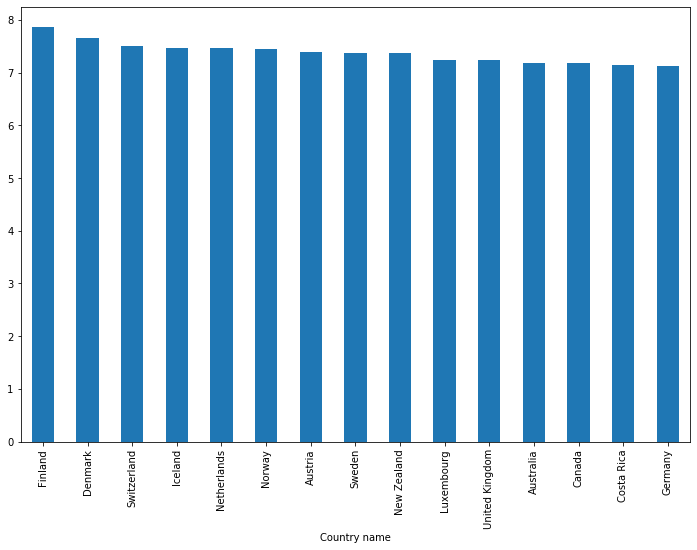In :
np.exp(data[
data['Year'] == 2018
].groupby('Continent')['Log GDP per capita']\
.mean()).sort_values().plot(
kind='barh',
figsize=(12,8)
)

Out:
<matplotlib.axes._subplots.AxesSubplot at 0x130e24080>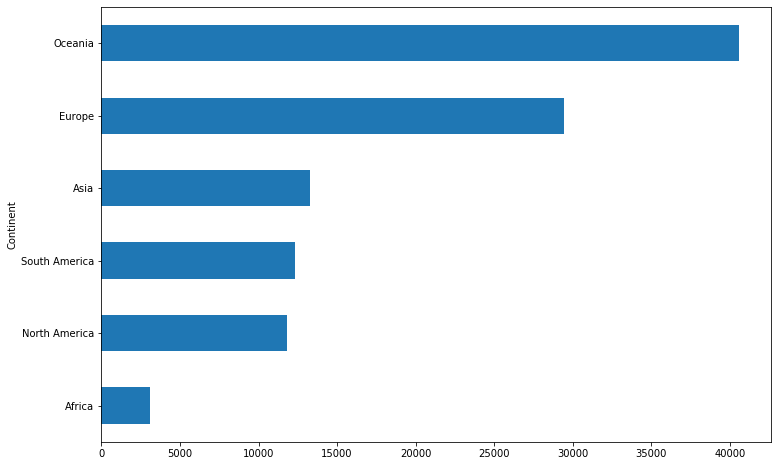In :
data['Life Ladder'].plot(
kind='box',
figsize=(12,8)
)

Out:
<matplotlib.axes._subplots.AxesSubplot at 0x13117af60>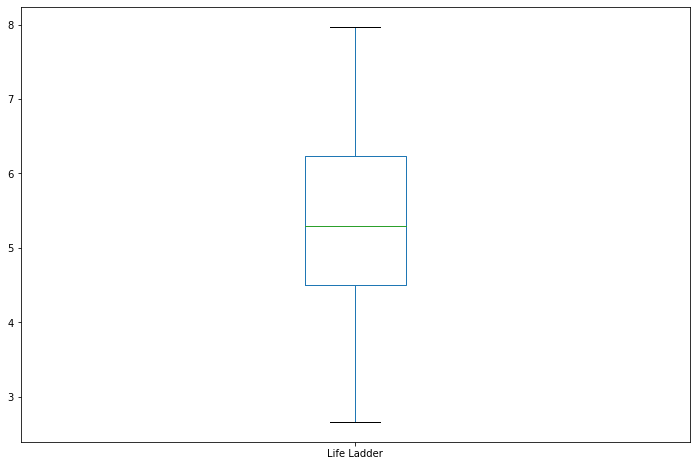In :
data[['Healthy life expectancy at birth','Gapminder Life Expectancy']].plot(
kind='scatter',
x='Healthy life expectancy at birth',
y='Gapminder Life Expectancy',
figsize=(12,8)
)

Out:
<matplotlib.axes._subplots.AxesSubplot at 0x13152a080>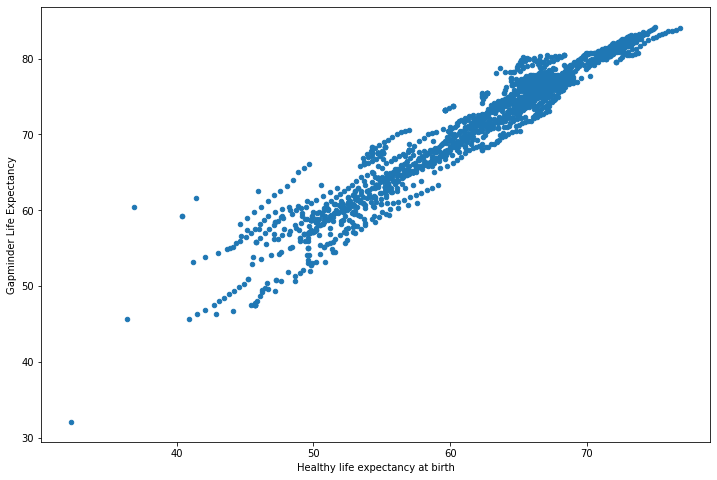In :
data[data['Year'] == 2018].plot(
kind='hexbin',
x='Healthy life expectancy at birth',
y='Generosity',
gridsize=20,
figsize=(12,8),
cmap="Blues", # defaults to greenish
sharex=False # required to get rid of a bug
)

Out:
<matplotlib.axes._subplots.AxesSubplot at 0x130f6bb70>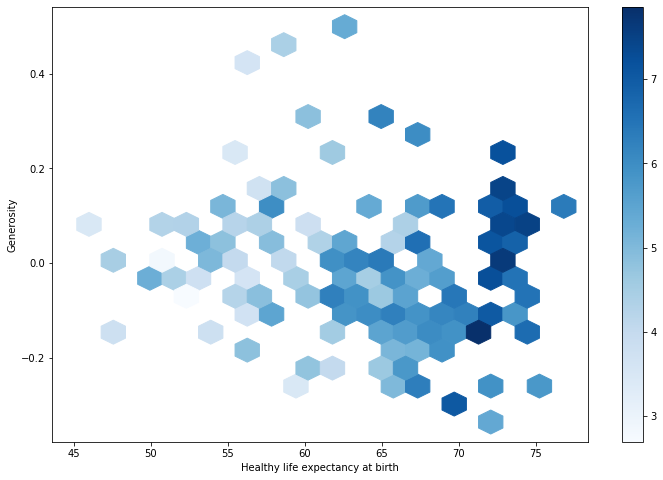In :
data[data['Year'] == 2018].groupby(
['Continent']
)['Gapminder Population'].sum().plot(
kind='pie',
figsize=(16,10),
cmap="Blues_r", # defaults to orange
)

Out:
<matplotlib.axes._subplots.AxesSubplot at 0x13341f7b8>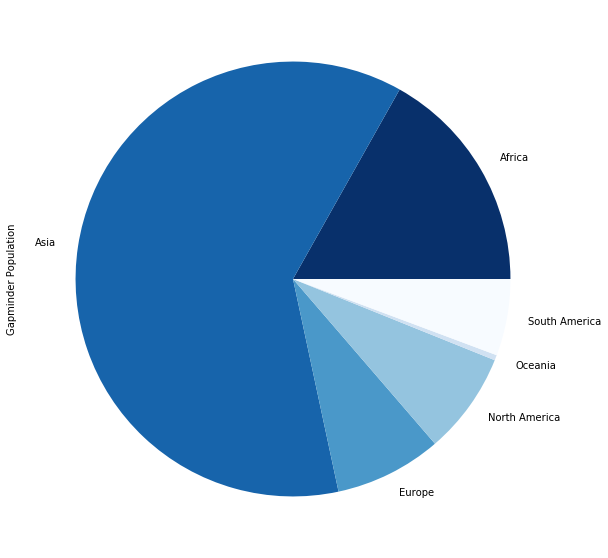In :
data.groupby(
['Year','Continent']
)['Gapminder Population'].sum().unstack().plot(
kind='area',
figsize=(12,8),
cmap="Blues", # defaults to orangish
)

Out:
<matplotlib.axes._subplots.AxesSubplot at 0x133460a20>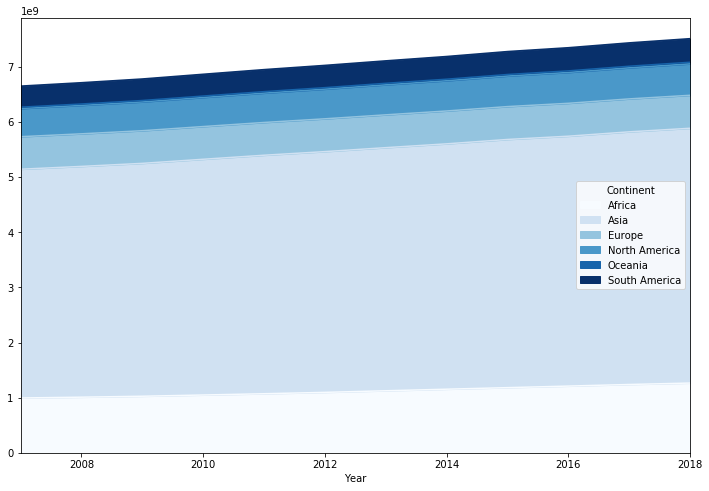In :
data[
data['Country name'] == 'Germany'
kind='line',
figsize=(12,8)
)

Out:
<matplotlib.axes._subplots.AxesSubplot at 0x1335ced68>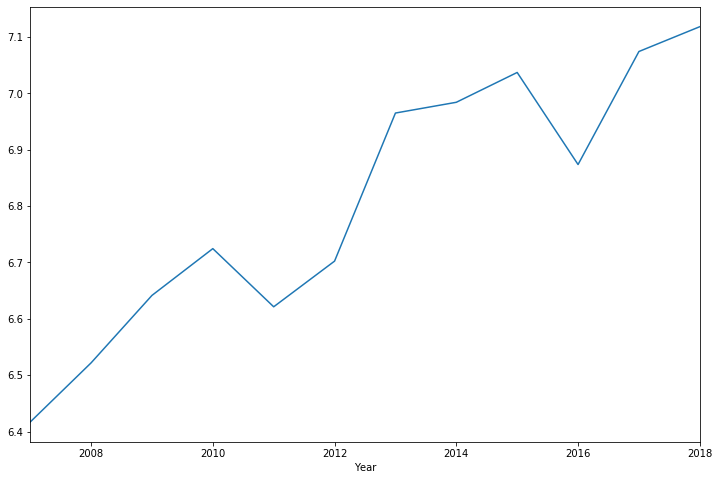# Seaborn¶

## Plotting univariate distributions¶

As mentioned before, I am a big fan of distributions. Histograms and Kernel density alike are potent ways of visualizing a the key features of a particular variable. Let's look at how we generate distributions for

In :
sns.reset_defaults()

In :
sns.set(
style="white",
palette="muted" # prettier colors
)

In :
sns_data = data[
(data['Year'] == 2018) &
(data['Continent'] == 'Asia')
]

sns.distplot(
)

sns.despine() # pretty graphs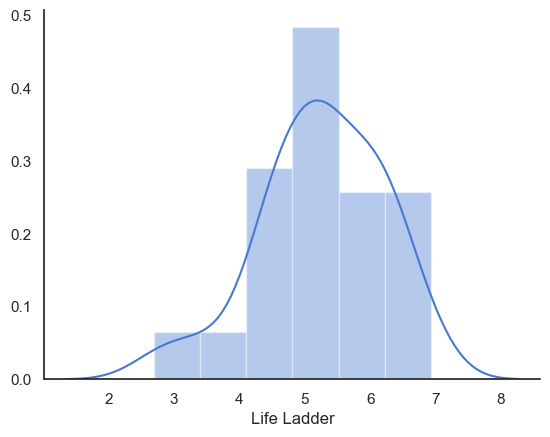In :
__sns_data = {}
for val in data['Mean Log GDP per capita'].cat.categories:
__sns_data[val] = data[
(data['Year'] == 2018) &
(data['Mean Log GDP per capita'] == val)
]

sns.kdeplot(
label=val
)

sns.despine()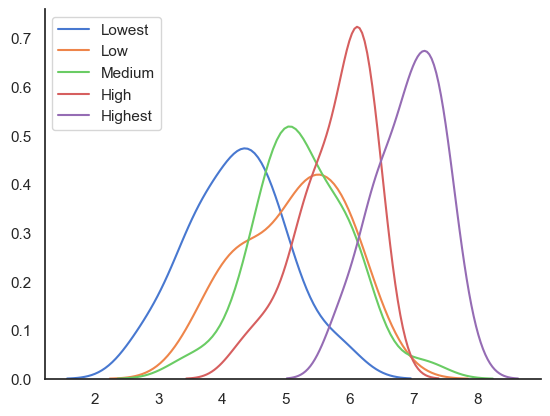## Plotting bivariate distributions¶

Whenever I want to visualy explore the relationship between two or multiple variables it typically comes down to some form of scatterplot and an assessment of joint distributions. There are three variations of a conceptually similar plot, where a in the center graph a form of joint distribution is shown and at the right and top side of the center graph the marginal distributions are depicted.

In :
sns.reset_defaults()
sns.set(
rc={'figure.figsize':(7,5)},
style="white"
)

In :
sns.jointplot(
x='Log GDP per capita',
data=data,
kind='scatter'
)

Out:
<seaborn.axisgrid.JointGrid at 0x134fb22e8>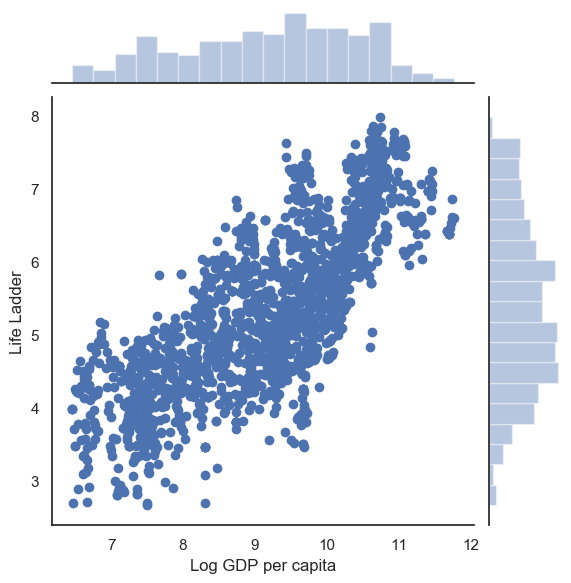In :
sns.jointplot(
x='Log GDP per capita',
data=data,
kind='kde'
)

Out:
<seaborn.axisgrid.JointGrid at 0x13534abe0>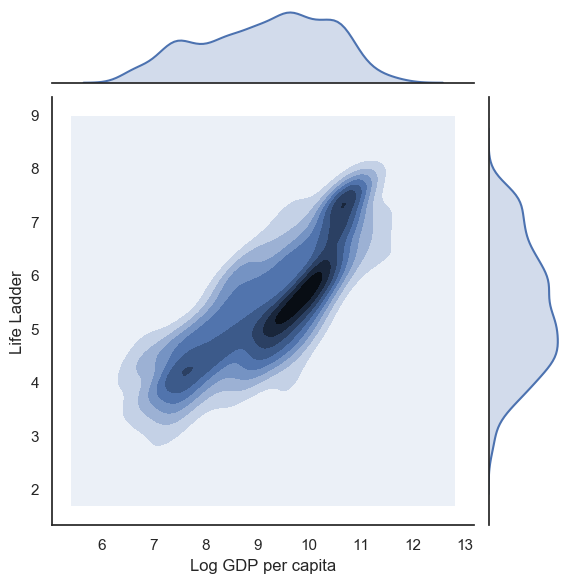In :
sns.jointplot(
x='Log GDP per capita',
data=data,
kind='hex'
)

Out:
<seaborn.axisgrid.JointGrid at 0x134decf98>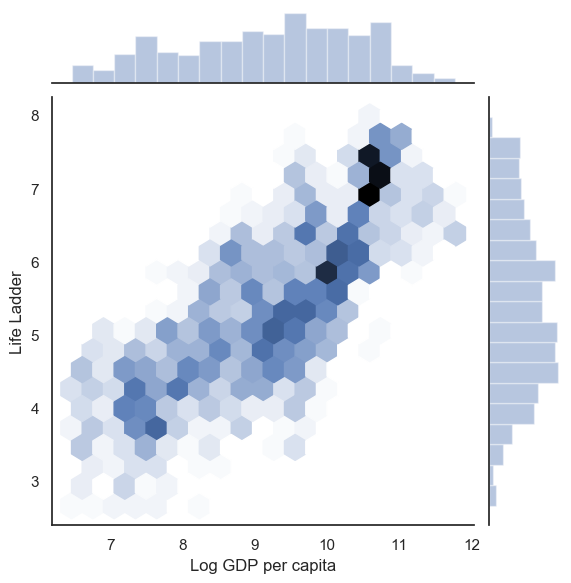In :
sns.scatterplot(
x='Log GDP per capita',
hue='Continent',
data=data[data['Year'] == 2018],
)

sns.despine()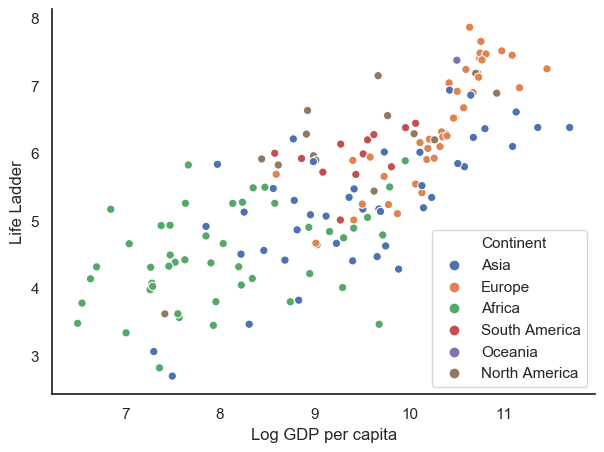In :
sns.scatterplot(
x='Log GDP per capita',
hue='Continent',
data=data[data['Year'] == 2018],
size='Gapminder Population'
)

sns.despine()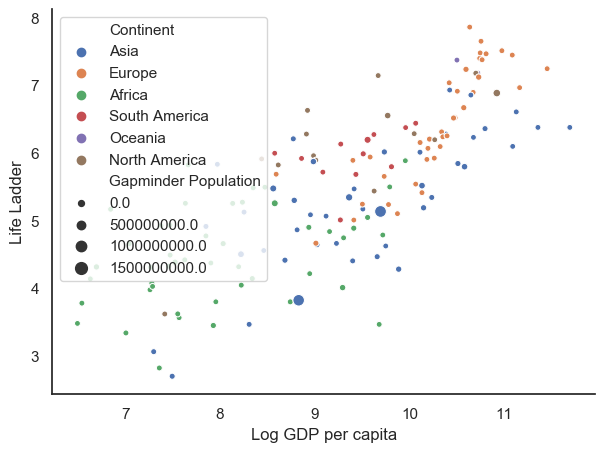In :
sns.set(
rc={'figure.figsize':(18,6)},
style="white"
)

sns.violinplot(
x='Continent',
hue='Mean Log GDP per capita',
data=data
)

sns.despine()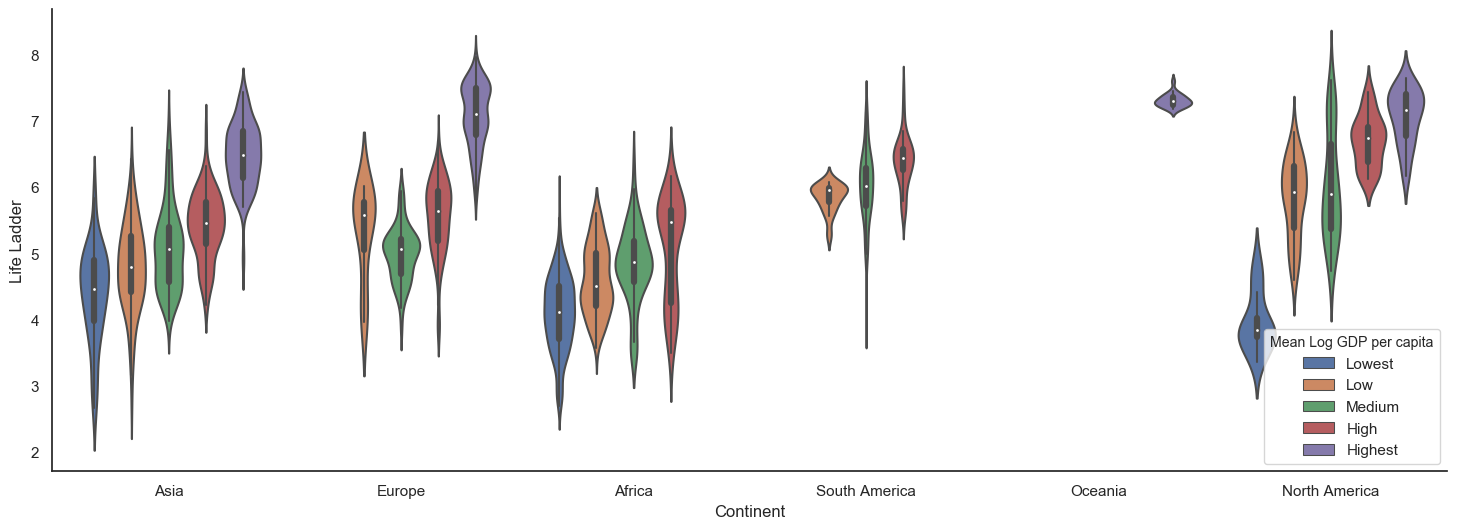In :
sns.set(
style="white",
palette="muted",
color_codes=True
)

g = sns.pairplot(
data[data.Year == 2018][[
'Life Ladder','Log GDP per capita',
'Social support','Healthy life expectancy at birth',
'Freedom to make life choices','Generosity',
'Perceptions of corruption', 'Positive affect','Negative affect',
'Confidence in national government',"Mean Log GDP per capita"]].dropna(),
hue="Mean Log GDP per capita"
)

g.fig.savefig('yolo.png')